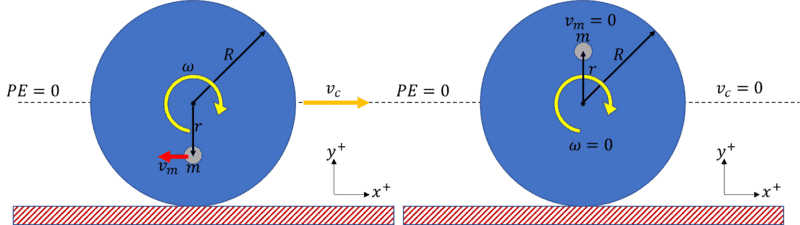# Rolling coin with point mass

• pandafish
In summary, the conversation discusses the energy and velocity of a rolling coin, with a focus on the minimum and maximum velocities that allow the coin to continue rolling without slipping. The point mass of the coin is shown to experience changes in kinetic energy as it moves along the table, with the maximum velocity potentially causing the coin to lift off the ground. There is also a discussion on the forces involved in the scenario, with different frames of reference and equations being options for analysis.f

#### pandafish

Homework Statement
A coin of radius R and mass M has a point mass m fixed at a distance r from its
centre. Find the minimum and maximum velocities which the coin can roll along the
table.
Relevant Equations
Conservation of energy: the difference in kinetic energy when the point mass is at its highest point and when it is at its lowest is equal to the difference in gravitational potential energy.

$$\frac{1}{2}m_0v^2 - \frac{1}{2}m_1v^2 = 2mgr$$
I reasoned that at the coin's slowest velocity, the energy it has must just be enough for it to reach the highest potential configuration: when the point mass is directly above the centre of mass of the coin, and its GPE is ##mg(R+r)##. I used this to find the minimum velocity, but I can't think of a reason there would be a max velocity for the coin.

...
I reasoned that at the coin's slowest velocity, the energy it has must just be enough for it to reach the highest potential configuration
What happens to its rotational velocity while that happens?

The rotational velocity decreases until it is 0, where the point mass is at the highest point.

The rotational velocity decreases, but not necessarily must reach 0, where the point mass is at the highest point.
The statement shows us that the coin rolls along the table.

Once the mass passes its highest point, can you see the same force that slowed the coin down inducing the rotational velocity to increase?

Yes, gravitational force on the mass creates a torque that increases and decreases the rotational velocity as the coin rolls.

Excellent!
The mass gains and loses potential energy in a cyclic manner.
Then, what locations of the mass correspond to the minimum and maximum rotational velocity (or kinetic energy) of the coin as it rolls along the table?

When the mass is furthest from the table, the kinetic energy is lowest, and when the mass is closest to the table, the kinetic energy is greatest?

•Lnewqban
Exactly!

•pandafish
Oh, I think I understand now. Thank you

•LnewqbanAren't they asking what is the minimum velocity ##v_c## that if the coin rolls without slipping it can continue to roll?

I can't see why there should be a maximum velocity though...Last edited:
I can't see why there should be a maximum velocity though...At some velocity the coin will lift clear of the ground.

•pandafish, erobz and Lnewqban
View attachment 324387

Aren't they asking what is the minimum velocity ##v_c## that if the coin rolls without slipping it can continue to roll?

I can't see why there should be a maximum velocity though...Yes, that is what I originally assumed the problem to be asking.

At some velocity the coin will lift clear of the ground.
Does this mean when the centripetal force on the point mass balances out its weight force, the coin would no longer be rolling?

Does this mean when the centripetal force on the point mass balances out its weight force, the coin would no longer be rolling?
Be careful. The coin as a whole has to lift clear of the ground.

Be careful. The coin as a whole has to lift clear of the ground.
Just a clarification: Its a centrifugal force in the rotating frame of the coin that the point mass is feeling that balances the total weight, not a centripetal force , correct?

Just a clarification: Its a centrifugal force in the rotating frame of the coin that the point mass is feeling that balances the total weight, not a centripetal force , correct?
One can use centrifugal force, centripetal force or centripetal acceleration in a description of the scenario. It depends on what frame of reference you choose to adopt and what balance equation you choose to write down.

But yes I agree that one possible balance would be centrifugal force versus gravitational force.

•erobz